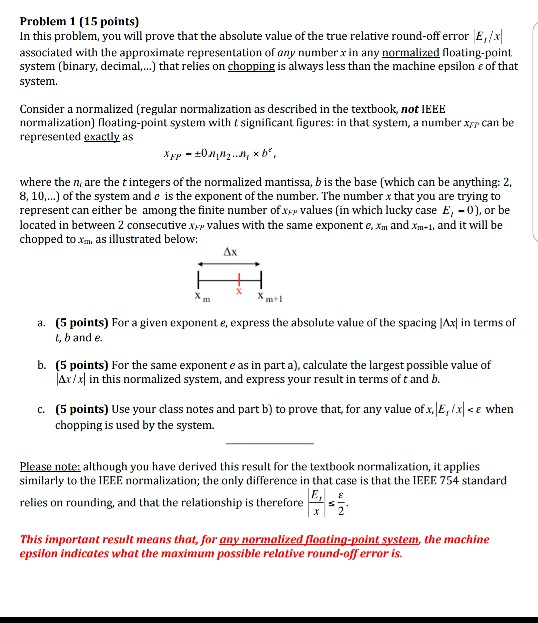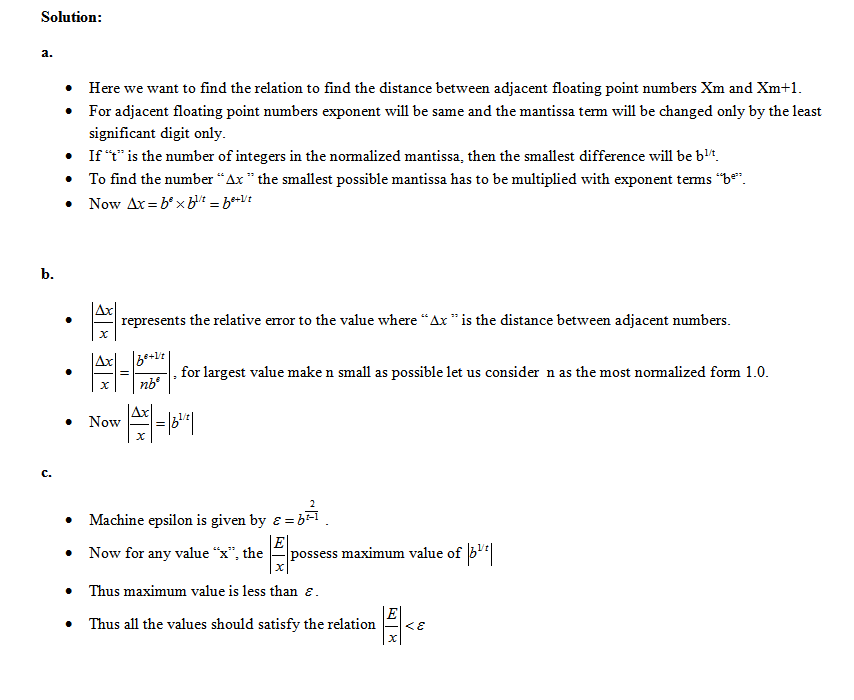# Question & Answer: /x| associated with the approximate representation of any number x in any no…..In this problem, you will prove that the absolute value of the true relative round-off error |E_I/x| associated with the approximate representation of any number x in any normalized floating-point system(binary, decimal ….) that relies on chopping is always less than the machine epsilon epsilon of that system. Consider a normalized (regular normalization as described in the textbook, not IEEE normalization) floating-point system with t significant figures: in that system, a number x_FP can be represented exactly as x_FP = plusminus 0 n_1, n_2 … n_ times b^where the n_ are the t integers of the normalized mantissa, b is the base (which can be anything: 2, 8, 10, ..) of the system and e is the exponent of the number. The number x that you are trying to represent can either be among the finite number of x_FP values (in which lucky case E_ = 0), or be located in between 2 consecutive x_FP values with the same exponent e, x_m and x_m + 1, and it will be chopped to x_m, as illustrated below: a. For a given exponent e, express the absolute value of the spacing |delta x| in terms of t, b and e. b. For the same exponent e as in part a), calculate the largest possible value of |delta x/x| in this normalized system, and express your result in terms of t and b. c. Use your class notes and part b) to prove that, for any value of x, |E_I/x|